Hard core Yukawa potential

The hard core Yukawa potential  has two forms, the attractive Yukawa potential: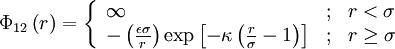$\Phi_{12}\left( r \right) = \left\{ \begin{array}{lll} \infty & ; & r < \sigma \\ - \left( \frac{ \epsilon \sigma }{r}\right) \exp \left[- \kappa \left( \frac{r}{\sigma} - 1 \right) \right] & ; & r \ge \sigma \end{array} \right.$

and the repulsive form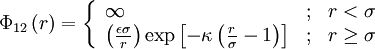$\Phi_{12}\left( r \right) = \left\{ \begin{array}{lll} \infty & ; & r < \sigma \\ \left( \frac{ \epsilon \sigma }{r}\right) \exp \left[- \kappa \left( \frac{r}{\sigma} - 1 \right) \right] & ; & r \ge \sigma \end{array} \right.$

where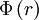$\Phi\left( r \right)$ is the intermolecular pair potential,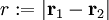$r := |\mathbf{r}_1 - \mathbf{r}_2|$ is the distance between site 1 and site 2,$\sigma$ is the hard diameter,$\epsilon$ is the energy well depth (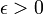$\epsilon > 0$), and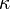$\kappa$ is a parameter that controls the interaction range (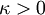$\kappa > 0$).

The repulsive form has been used to study charge-stabilised colloid-colloid interactions.

Critical point

For the attractive form of the potential, from a study of the law of corresponding states, one has (Eq. 3 in )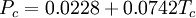$P_c = 0.0228 + 0.0742 T_c$

and (Eq. 4)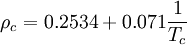$\rho_c = 0.2534 + 0.071 \frac{1}{T_c}$

The repulsive form of the potential has no critical point.

Triple points

The triple points for this model have been studied by Azhar and co-workers .

Virial coefficients

For the attractive form of the potential the virial coefficients have been calculated by Naresh and Singh .